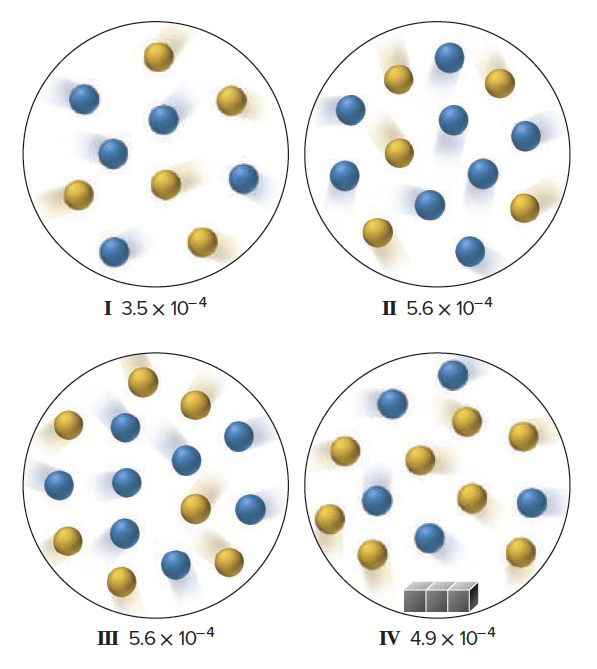# Problem: The scenes depict four initial reaction mixtures for the reaction of A (blue) and B (yellow), with and without a solid present (gray cubes). The initial rate, –d[A]/dt (in mol/L·s), is shown, with each sphere representing 0.010 mol and the container volume at 0.50 L.Do the gray cubes have a catalytic effect? Explain.

###### FREE Expert Solution

We’re being asked to determine if the gray cubes have a catalytic effect.

We're given the initial rates and the amount of reactant in each reaction.

Rate is equal to:

$\overline{){\mathbf{rate}}{\mathbf{=}}{\mathbf{-}}\frac{\mathbf{∆}\mathbf{\left[}\mathbf{A}\mathbf{\right]}}{\mathbf{∆}\mathbf{t}}}$

We have to compare the time for each reaction. First, let's calculate the initial amount of A and B in each mixture:

$\overline{){\mathbf{molarity}}{\mathbf{\left(}}{\mathbf{M}}{\mathbf{\right)}}{\mathbf{=}}\frac{\mathbf{mol}}{\mathbf{L}}}$

Mixture I:

molarity A = 0.1 M

molarity B = 0.1 M

Mixture II:

molarity A = 0.16 M

95% (16 ratings)###### Problem Details

The scenes depict four initial reaction mixtures for the reaction of A (blue) and B (yellow), with and without a solid present (gray cubes). The initial rate, –d[A]/dt (in mol/L·s), is shown, with each sphere representing 0.010 mol and the container volume at 0.50 L.Do the gray cubes have a catalytic effect? Explain.

Frequently Asked Questions

What scientific concept do you need to know in order to solve this problem?

Our tutors have indicated that to solve this problem you will need to apply the Catalyst concept. If you need more Catalyst practice, you can also practice Catalyst practice problems.

What professor is this problem relevant for?

Based on our data, we think this problem is relevant for Professor Savizky's class at COLUMBIA.

What textbook is this problem found in?

Our data indicates that this problem or a close variation was asked in Chemistry: The Molecular Nature of Matter and Change - Silberberg 8th Edition. You can also practice Chemistry: The Molecular Nature of Matter and Change - Silberberg 8th Edition practice problems.